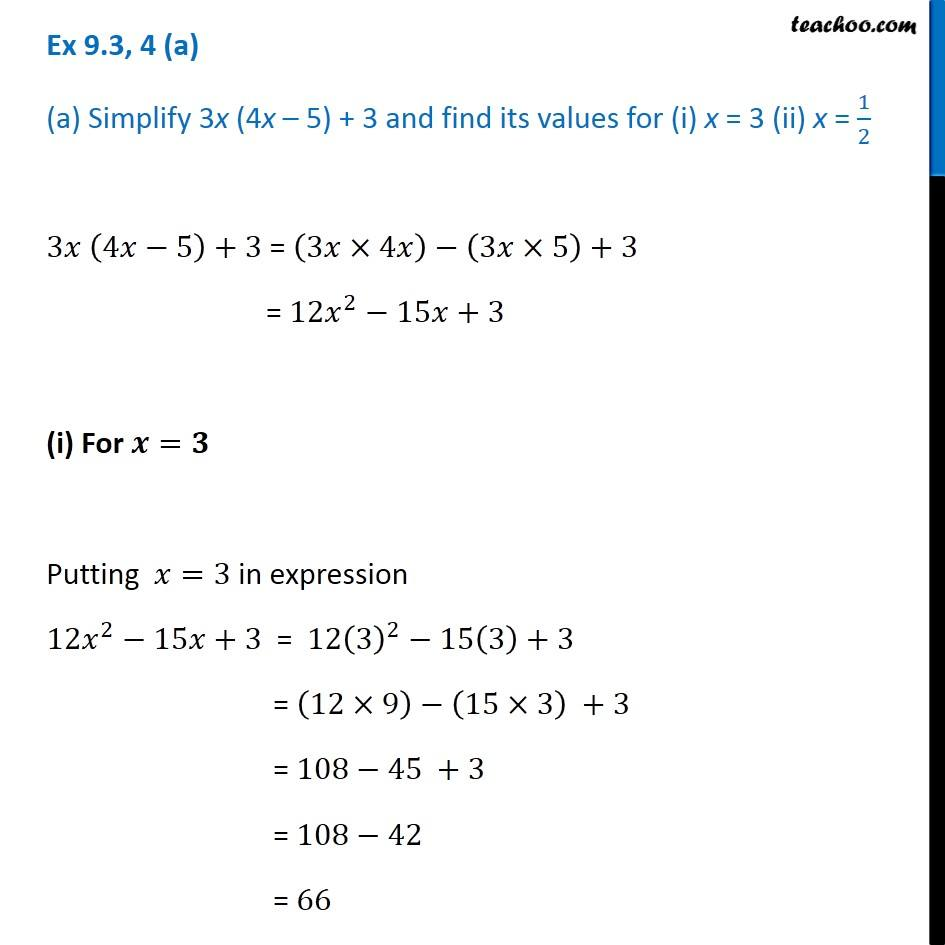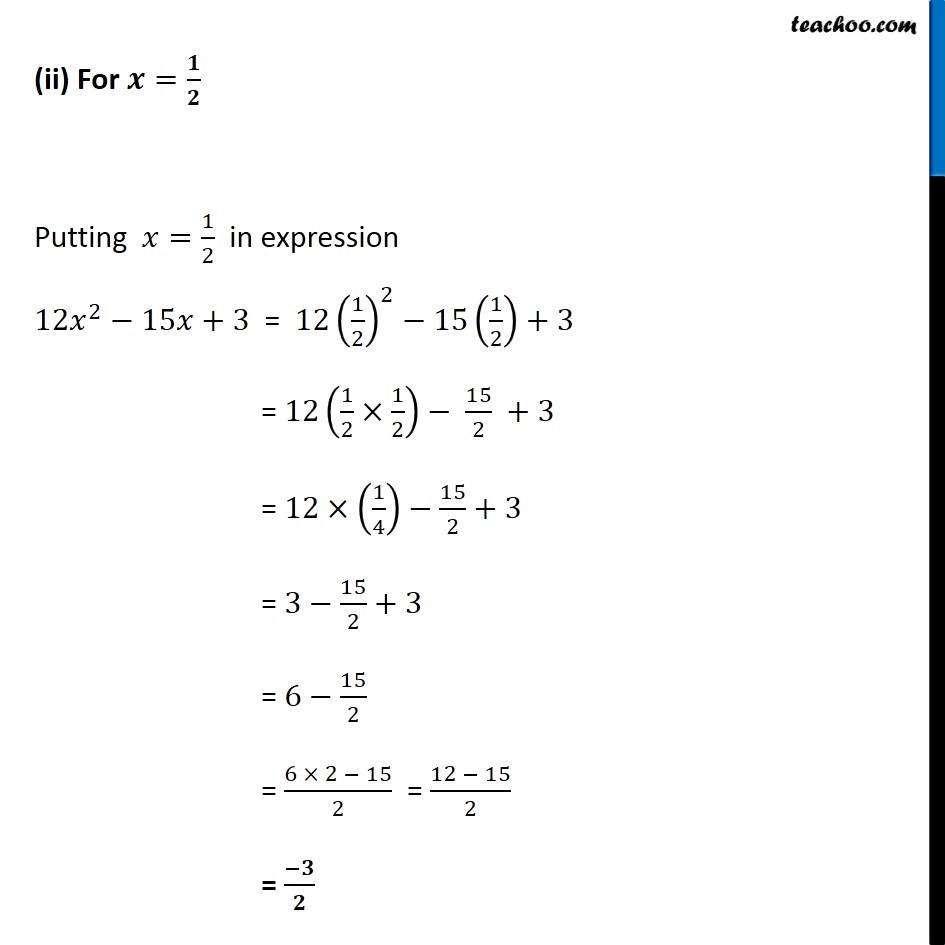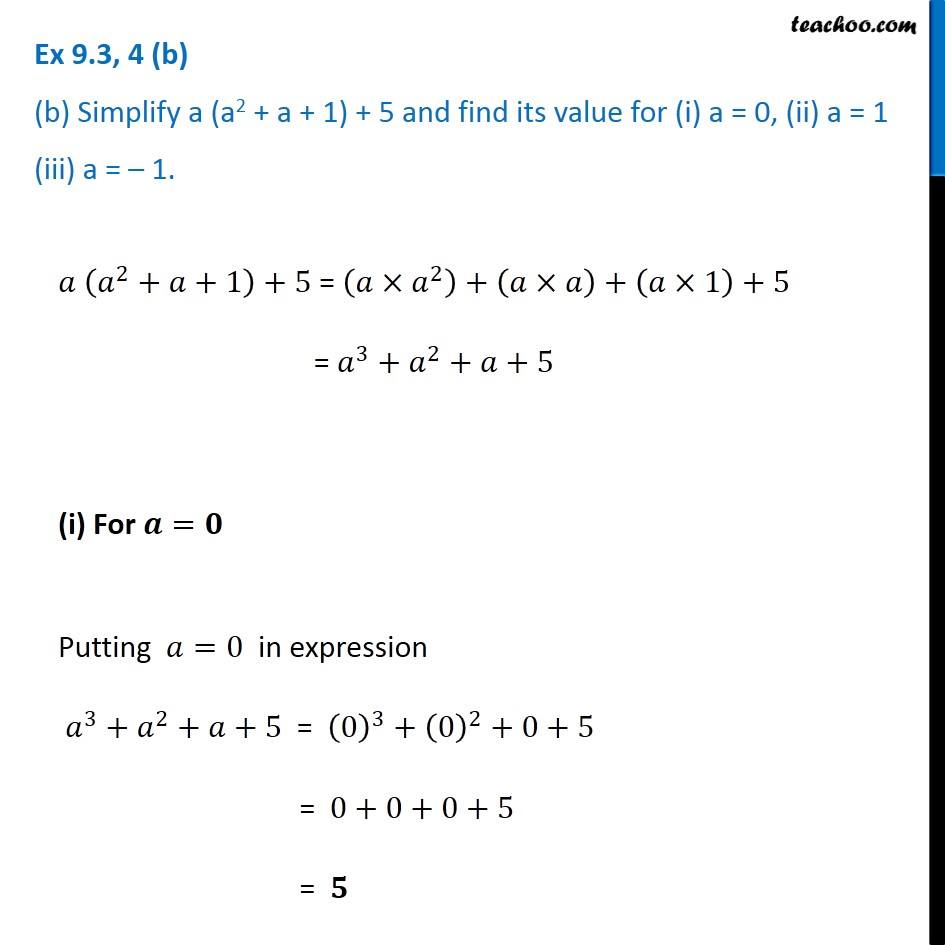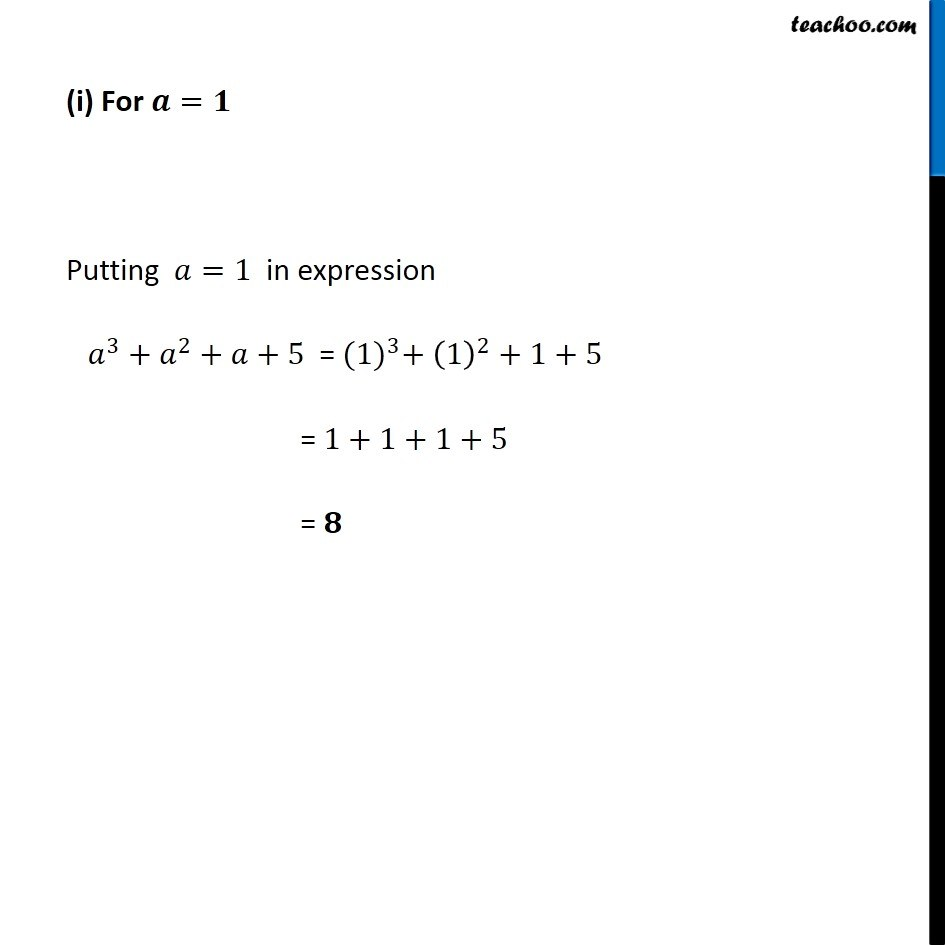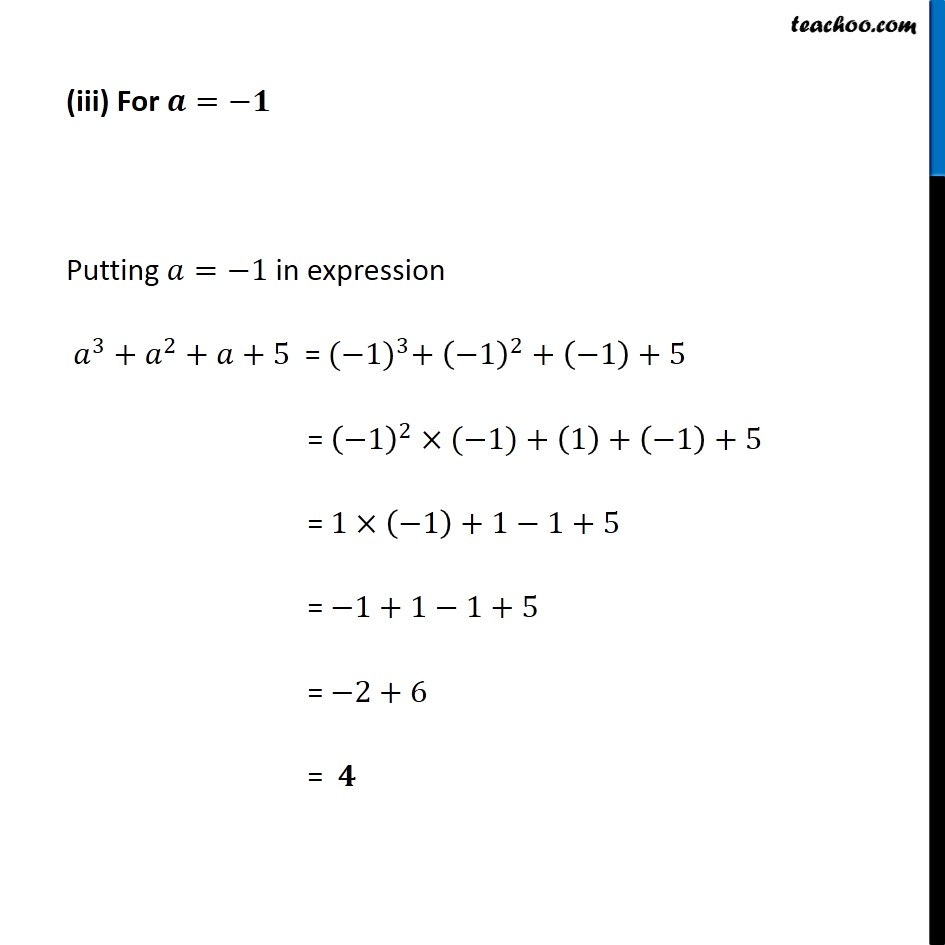Subscribe to our Youtube Channel - https://you.tube/teachoo

1. Chapter 9 Class 8 Algebraic Expressions and Identities
2. Serial order wise
3. Ex 9.3

Transcript

Ex 9.3, 4 (a) (a) Simplify 3x (4x – 5) + 3 and find its values for (i) x = 3 (ii) x = 1/2 3𝑥 (4𝑥−5)+3 = (3𝑥×4𝑥)−(3𝑥×5)+3 = 12𝑥^2−15𝑥+3 (i) For 𝒙=𝟑 Putting 𝑥=3 in expression 12𝑥^2−15𝑥+3 = 12(3)^2−15(3)+3 = (12×9)−(15×3) +3 = 108−45 +3 = 108−42 = 66 (ii) For 𝒙=𝟏/𝟐 Putting 𝑥=1/2 in expression 12𝑥^2−15𝑥+3 = 12(1/2)^2−15(1/2)+3 = 12(1/2×1/2)− 15/2 +3 = 12×(1/4)−15/2+3 = 3−15/2+3 = 6−15/2 = (6 × 2 − 15)/2 = (12 − 15)/2 = (−𝟑)/𝟐 Ex 9.3, 4 (b) (b) Simplify a (a2 + a + 1) + 5 and find its value for (i) a = 0, (ii) a = 1 (iii) a = – 1. 𝑎 (𝑎^2+𝑎+1)+5 = (𝑎×𝑎^2 )+(𝑎×𝑎)+(𝑎×1)+5 = 𝑎^3+𝑎^2+𝑎+5 (i) For 𝒂=𝟎 Putting 𝑎=0 in expression 𝑎^3+𝑎^2+𝑎+5 = (0)^3+(0)^2+0+5 = 0+0+0+5 = 𝟓 (i) For 𝒂=𝟏 Putting 𝑎=1 in expression 𝑎^3+𝑎^2+𝑎+5 = 〖(1)〗^3+ (1)^2+1+5 = 1+1+1+5 = 𝟖 (iii) For 𝒂=−𝟏 Putting 𝑎=−1 in expression 𝑎^3+𝑎^2+𝑎+5 = 〖(−1)〗^3+ (−1)^2+(−1)+5 = (−1)^2×(−1)+(1)+(−1)+5 = 1×(−1)+1−1+5 = −1+1−1+5 = −2+6 = 𝟒

Ex 9.3